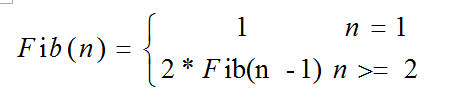# [剑指Offer]2.变态跳台阶/*---------------------------------------
*   日期：2015-07-19
*   作者：SJF0115
*   题目: 2.变态跳台阶
*   网址：http://www.nowcoder.com/books/coding-interviews/22243d016f6b47f2a6928b4313c85387?rp=1
*   结果：AC
*   来源：剑指Offer
*   博客：
-----------------------------------------*/
#include <iostream>
using namespace std;

class Solution {
public:
int jumpFloorII(int number) {
if(number <= 0){
return 0;
}//if
else if(number == 1){
return 1;
}//else
return 2*jumpFloorII(number - 1);
}
};

int main(){
Solution s;
int number = 5;
cout<<s.jumpFloorII(number)<<endl;
return 0;
}

<script type="text/javascript"> $(function () {$('pre.prettyprint code').each(function () { var lines = $(this).text().split('\n').length; var$numbering = $('<ul/>').addClass('pre-numbering').hide();$(this).addClass('has-numbering').parent().append($numbering); for (i = 1; i <= lines; i++) {$numbering.append($('<li/>').text(i)); };$numbering.fadeIn(1700); }); }); </script>